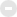day = new Date( ) nge_Hour = day.getHours( ) var nge_warmprompt = ""; if (nge_Hour == 0) nge_warmprompt = "现在已经过凌晨了，身体是无价的资本喔，早点休息吧！" if (nge_Hour == 1) nge_warmprompt = "凌晨1点多了，工作是永远都做不完的，别熬坏身子！" if (nge_Hour == 2) nge_warmprompt = "该休息了，身体可是革命的本钱啊！" if (nge_Hour == 3) nge_warmprompt = "夜深了，熬夜很容易导致身体内分泌失调，长痘痘的！" if (nge_Hour == 4) nge_warmprompt = "四点过了，你明天不上班？？？" if (nge_Hour == 5) nge_warmprompt = "你知道吗，此时是国内网络速度最快的时候！" if ((nge_Hour == 9) || (nge_Hour ==10)) nge_warmprompt = "上午好！今天你看上去好精神哦！" if (( nge_Hour == 11) || (nge_Hour == 12)) nge_warmprompt = "该吃午饭啦！有什么好吃的？你有中午休息的好习惯吗？" if (( nge_Hour >= 13) && (nge_Hour <= 17)) nge_warmprompt = "下午好！外面的天气好吗？记得朵朵白云曾捎来朋友殷殷的祝福。" if (( nge_Hour >= 17) && (nge_Hour <= 18)) nge_warmprompt = "太阳落山了！快看看夕阳吧！如果外面下雨，就不必了 ^_^" if (( nge_Hour >= 18) && (nge_Hour <= 19)) nge_warmprompt = "晚上好，今天的心情怎么样，来论坛和我们诉说吧！" if (( nge_Hour >= 19) && (nge_Hour <= 21)) nge_warmprompt = "忙碌了一天，累了吧？来看看帖子，放松下吧！" if (( nge_Hour >= 22) && (nge_Hour <= 23)) nge_warmprompt = "这么晚了，还在上网？早点洗洗睡吧，睡前记得洗洗脸喔！" document.write("<font color='#2iE9AFE'><a href='//www.guhei.net/member.php?mod=logging&action=login&referer'>「登陆后享受更多权益~」 </a>主上，") document.write(nge_warmprompt) document.write("</font>")计算机网络计算机

104 / 583移动终端

36 / 252硬件相关

95 / 513

GMT+8, 2019-6-25 11:42 , Processed in 0.146741 second(s), 24 queries , Redis On.